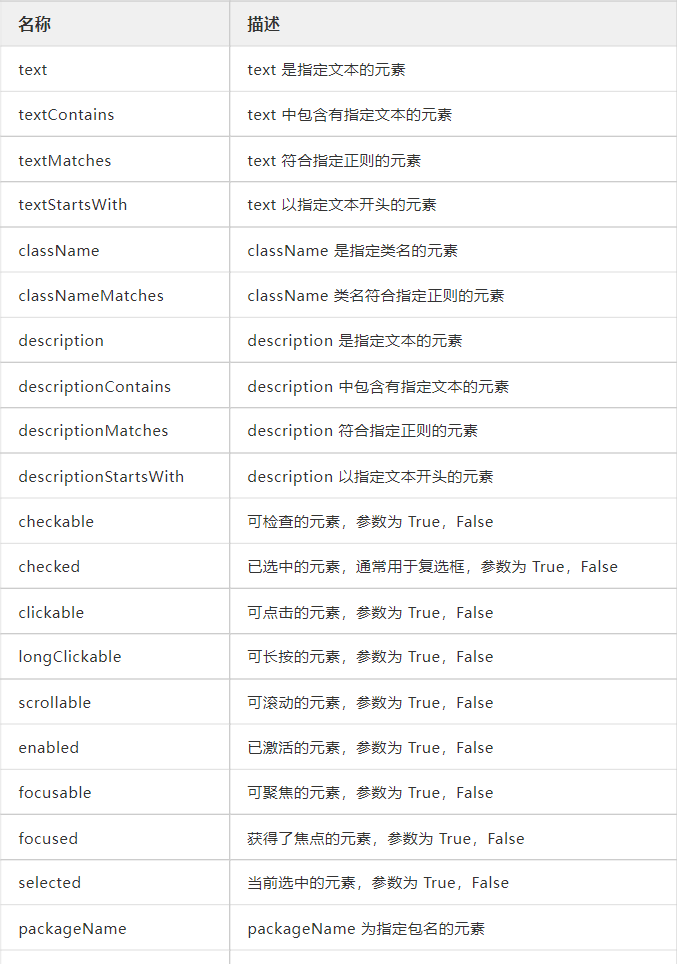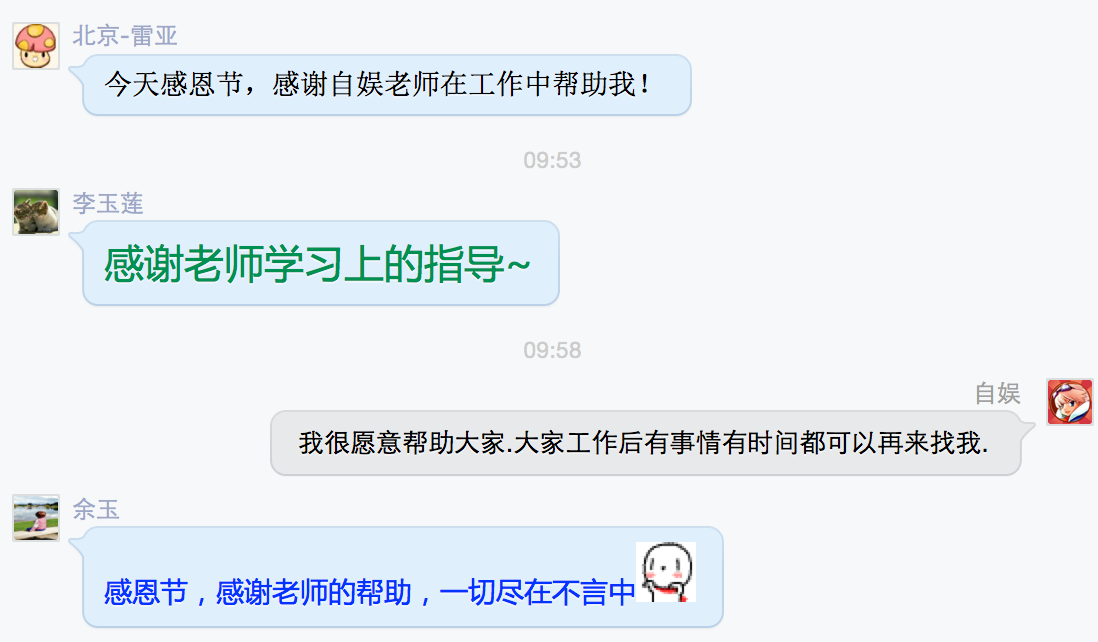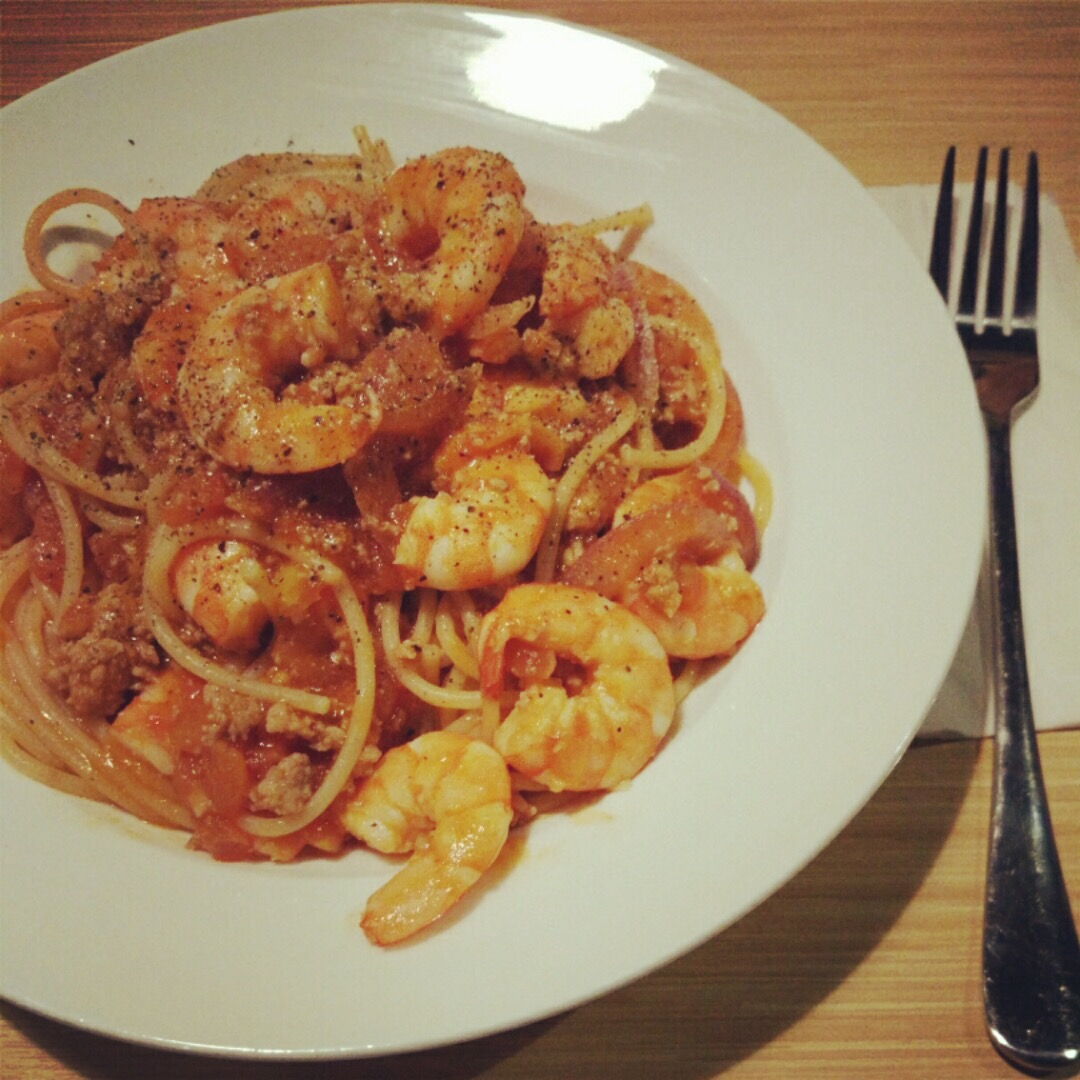# 高效使用Python可视化工具Matplotlib

+关注继续查看

Matplotlib是Python中最常用的可视化工具之一,可以非常方便地创建海量类型的2D图表和一些基本的3D图表。本文主要介绍了在学习Matplotlib时面临的一些挑战，为什么要使用Matplotlib，并推荐了一个学习使用Matplotlib的步骤。

matplotlib的新用户应该学习使用面向对象的接口。

matplotlib的另一个历史性挑战是，一些默认风格选项相当没有吸引力。 在R语言世界里，可以用ggplot生成一些相当酷的绘图，相比之下，matplotlib的选项看起来有点丑。令人欣慰的是matplotlib 2.0具有更美观的样式，以及非常便捷对可视化的内容进行主题化的能力。

1. 学习基本的matplotlib术语，尤其是什么是图和坐标轴
2. 始终使用面向对象的接口，从一开始就养成使用它的习惯
3. 用基础的pandas绘图开始你的可视化学习
4. 用seaborn进行更复杂的统计可视化
5. 用matplotlib来定制pandas或者seaborn可视化1. import pandas as pd
2.
3. import matplotlib.pyplot as plt
4.
5. from matplotlib.ticker import FuncFormatter
6.
7.
8.
10.1. top_10 = (df.groupby('name')['ext price''quantity'].agg({'ext price''sum''quantity''count'})
2.
3. .sort_values(by='ext price', ascending=False))[:10].reset_index()
4.
5. top_10.rename(columns={'name''Name''ext price''Sales''quantity''Purchases'}, inplace=True)1. plt.style.available

1. ['seaborn-dark'
2.
3. 'seaborn-dark-palette'
4.
5. 'fivethirtyeight'
6.
7. 'seaborn-whitegrid'
8.
9. 'seaborn-darkgrid'
10.
11. 'seaborn'
12.
13. 'bmh'
14.
15. 'classic'
16.
17. 'seaborn-colorblind'
18.
19. 'seaborn-muted'
20.
21. 'seaborn-white'
22.
23. 'seaborn-talk'
24.
25. 'grayscale'
26.
27. 'dark_background'
28.
29. 'seaborn-deep'
30.
31. 'seaborn-bright'
32.
33. 'ggplot'
34.
35. 'seaborn-paper'
36.
37. 'seaborn-notebook'
38.
39. 'seaborn-poster'
40.
41. 'seaborn-ticks'
42.
43. 'seaborn-pastel']

1. plt.style.use('ggplot'

1. top_10.plot(kind='barh', y="Sales", x="Name"1. fig, ax = plt.subplots()
2.
3. top_10.plot(kind='barh', y="Sales", x="Name", ax=ax)

1. fig, ax = plt.subplots()
2.
3. top_10.plot(kind='barh', y="Sales", x="Name", ax=ax)
4.
5. ax.set_xlim([-10000, 140000])
6.
7. ax.set_xlabel('Total Revenue'
8.
9. ax.set_ylabel('Customer');1. fig, ax = plt.subplots()
2.
3. top_10.plot(kind='barh', y="Sales", x="Name", ax=ax)
4.
5. ax.set_xlim([-10000, 140000])
6.
7. ax.set(title='2014 Revenue', xlabel='Total Revenue', ylabel='Customer')1. fig, ax = plt.subplots(figsize=(5, 6))
2.
3. top_10.plot(kind='barh', y="Sales", x="Name", ax=ax)
4.
5. ax.set_xlim([-10000, 140000])
6.
7. ax.set(title='2014 Revenue', xlabel='Total Revenue'
8.
9. ax.legend().set_visible(False)1. def currency(x, pos):
2.
3.     'The two args are the value and tick position'
4.
5.     if x >= 1000000:
6.
7.         return '${:1.1f}M'.format(x*1e-6) 8. 9. return '${:1.0f}K'.format(x*1e-3)

1. fig, ax = plt.subplots()
2.
3. top_10.plot(kind='barh', y="Sales", x="Name", ax=ax)
4.
5. ax.set_xlim([-10000, 140000])
6.
7. ax.set(title='2014 Revenue', xlabel='Total Revenue', ylabel='Customer'
8.
9. formatter = FuncFormatter(currency)
10.
11. ax.xaxis.set_major_formatter(formatter)
12.
13. ax.legend().set_visible(False1. Create the figure and the axes
2.
3. fig, ax = plt.subplots()
4.
5.
6.
7. # Plot the data and get the averaged
8.
9. top_10.plot(kind='barh', y="Sales", x="Name", ax=ax)
10.
11. avg = top_10['Sales'].mean()
12.
13.
14.
15. Set limits and labels
16.
17. ax.set_xlim([-10000, 140000])
18.
19. ax.set(title='2014 Revenue', xlabel='Total Revenue', ylabel='Customer'
20.
21.
22.
23. Add a line for the average
24.
25. ax.axvline(x=avg, color='b', label='Average', linestyle='--', linewidth=1)
26.
27.
28.
29. # Annotate the new customers
30.
31. for cust in [3, 5, 8]:
32.
33.     ax.text(115000, cust, "New Customer"
34.
35.
36.
37. # Format the currency
38.
39. formatter = FuncFormatter(currency)
40.
41. ax.xaxis.set_major_formatter(formatter)
42.
43.
44.
45. # Hide the legend
46.
47. ax.legend().set_visible(False)1. fig, (ax0, ax1) = plt.subplots(nrows=1, ncols=2, sharey=True, figsize=(7, 4))

1. # Get the figure and the axes
2.
3. fig, (ax0, ax1) = plt.subplots(nrows=1,ncols=2, sharey=True, figsize=(7, 4))
4.
5. top_10.plot(kind='barh', y="Sales", x="Name", ax=ax0)
6.
7. ax0.set_xlim([-10000, 140000])
8.
9. ax0.set(title='Revenue', xlabel='Total Revenue', ylabel='Customers'
10.
11.
12.
13. # Plot the average as a vertical line
14.
15. avg = top_10['Sales'].mean()
16.
17. ax0.axvline(x=avg, color='b', label='Average', linestyle='--', linewidth=1)
18.
19.
20.
21. # Repeat for the unit plot
22.
23. top_10.plot(kind='barh', y="Purchases", x="Name", ax=ax1)
24.
25. avg = top_10['Purchases'].mean()
26.
27. ax1.set(title='Units', xlabel='Total Units', ylabel=''
28.
29. ax1.axvline(x=avg, color='b', label='Average', linestyle='--', linewidth=1)
30.
31.
32.
33. # Title the figure
34.
35. fig.suptitle('2014 Sales Analysis', fontsize=14, fontweight='bold');
36.
37.
38.
39. # Hide the legends
40.
41. ax1.legend().set_visible(False
42.
43. ax0.legend().set_visible(False)Matplotlib支持许多不同格式文件的保存。 你可以用fig.canvas.get_supported_filetypes()查看系统支持的格式：

1. fig.canvas.get_supported_filetypes()

1. {'eps''Encapsulated Postscript'
2.
3. 'jpeg''Joint Photographic Experts Group'
4.
5. 'jpg''Joint Photographic Experts Group'
6.
7. 'pdf''Portable Document Format'
8.
9. 'pgf''PGF code for LaTeX'
10.
11. 'png''Portable Network Graphics'
12.
13. 'ps''Postscript'
14.
15. 'raw''Raw RGBA bitmap'
16.
17. 'rgba''Raw RGBA bitmap'
18.
19. 'svg''Scalable Vector Graphics'
20.
21. 'svgz''Scalable Vector Graphics'
22.
23. 'tif''Tagged Image File Format'
24.
25. 'tiff''Tagged Image File Format'}

1. fig.savefig('sales.png', transparent=False, dpi=80, bbox_inches="tight"ArcGIS使用Python脚本工具

1230 0![](https://ceshiren.com/uploads/default/original/3X/f/a/facb6e29bf503b39571011963faf36c586c673c0.jpeg) Google 官方提供了一个 Android 自动化测试工具（Java 库），基于 Accessibility 服务，功能很强，可以对第三方 App 进行测试，获取屏幕上任意一个 App 的
106 0399 0SQLite数据库常用语句及MAC上的SQLite可视化工具MeasSQLlite使用（一）
SQLite数据库常用语句及MAC上的SQLite可视化工具MeasSQLlite使用
89 0I want a build rule to be triggered by an include directive if the target of the include is out of date or doesn't exist.
1018 0【译】使用C# yield关键字来提高性能和可读性
1073 0Bootstrap可视化布局系统使用文档
10304 0Uber开源数据可视化工具deck.gl获重大升级
1177 0【ZooKeeper Notes 25】ZooKeeper运维之使用SnapshotFormatter可视化快照数据
1353 0Qt编写数据可视化大屏界面电子看板10-改造QCustomPlot

1230 0

17112

2569

JS零基础入门教程（上册）